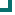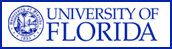Enterococcus faecium (SOUTHEAST Data) 2004 2005 2006 2007 2008 2009 2010 ampicillin 15.3%n=432 13.1%n=466 12.1%n=618 11.8%n=746 14.6%n=472 12.1%n=99 31.9%n=72 vancomycin 35.4%n=656 35%n=488 25.2%n=755 21.6%n=746 19.9%n=472 35.4%n=99 47.2%n=72 quinupristin/dalfopristin 100%n=99 72.7%n=22 99.1%n=111 47.3%n=207 96.3%n=27 nitrofurantoin 40.8%n=120 80.2%n=91 25.8%n=190 15.2%n=197 5.7%n=35 75%n=32 tetracycline 37%n=100 39.5%n=86 61.8%n=165 53.9%n=258 58.8%n=34 29.3%n=58 45.5%n=11 gentamicin 100%n=11 95.7%n=23 94.1%n=17 amikacin 7.7%n=26 chloramphenicol 95.7%n=23 93.3%n=15 rifampim 34.6%n=26 36.4%n=22 36.4%n=11 21.9%n=64 16.1%n=62 28.6%n=28 Totals 35.3%n=1456 31.8%n=1227 28.6%n=1850 24.6%n=2241 19.5%n=1030 32.9%n=377 38.3%n=183

This data is accurate as of: 11/22/2016 11:53:56 AM

Drugs with less than 10 isolates are removed from the chart and from the graph. The following drugs had some data removed: quinupristin/dalfopristin, chloramphenicol, rifampim, nitrofurantoin, tetracycline, gentamicin, amikacin, ampicillin, vancomycin.ARMProgram.com is a project run by the University of Florida. Copyright 2011. All Rights Reserved. Site by JMarc Technologies, LLC